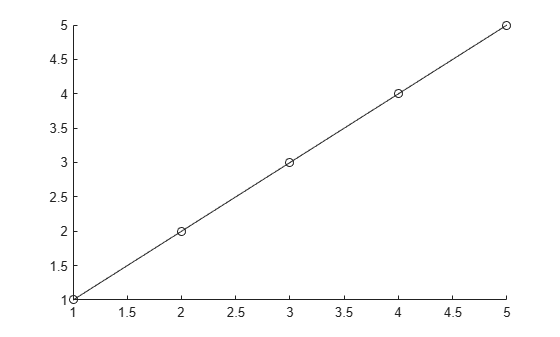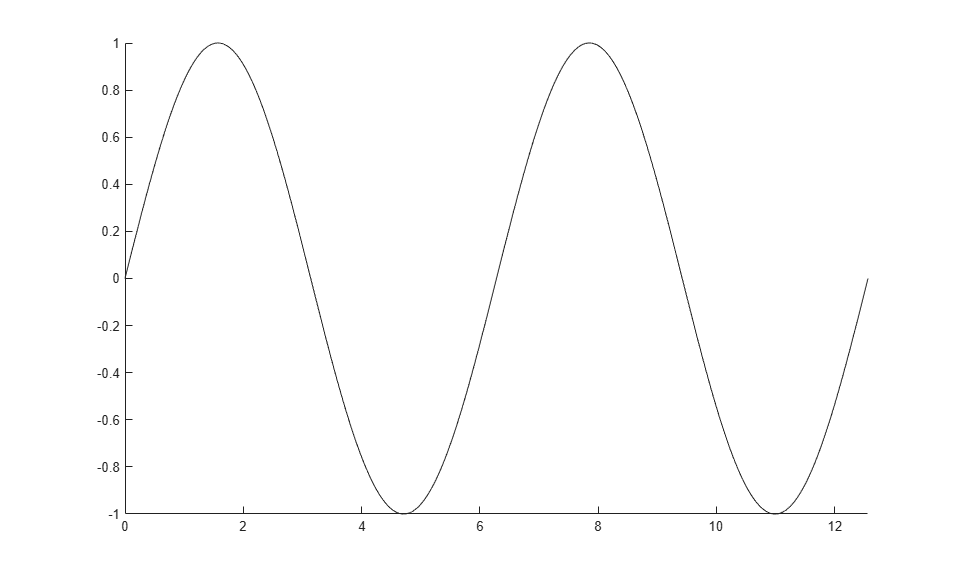## Syntax

``addpoints(an,x,y)``
``addpoints(an,x,y,z)``

## Description

example

````addpoints(an,x,y)` adds points defined by `x` and `y` to the animated line specified by `an`. Create an animated line with the `animatedline` function. To display the updates on the screen, use `drawnow` or ```drawnow limitrate```. New points automatically connect to previous points. ```
````addpoints(an,x,y,z)` adds points defined by `x`, `y`, and `z` to the 3-D animated line specified by `an`.```

## Examples

collapse all

Create an animated line object with no data. Then, add five points to the line. Use a circle to mark each point.

```h = animatedline('Marker','o'); x = 1:5; y = 1:5; addpoints(h,x,y)```Create an animated line using the `animatedline` function. Then, add points to the line within a loop to create an animation. Set the axis limits before the loop to prevent the limits from changing.

```figure h = animatedline; axis([0 4*pi -1 1]) for x = linspace(0,4*pi,10000) y = sin(x); addpoints(h,x,y) drawnow limitrate end ```## Input Arguments

collapse all

`AnimatedLine` object. Create an `AnimatedLine` object using the `animatedline` function.

First coordinate values, specified as a scalar or a vector. For Cartesian axes, the first coordinate is x-axis position. For geographic axes, the first coordinate is latitude in degrees.

The length of `x` must equal the length of `y`.

Example: `11:20`

Data Types: `double`

Second coordinate values, specified as a scalar or a vector. For Cartesian axes, the second coordinate is y-axis position. For geographic axes, the second coordinate is longitude in degrees.

The length of `y` must equal the length of `x`.

Example: `11:20`

Data Types: `double`

Third coordinate values, specified as a scalar or a vector. For Cartesian axes, the third coordinate is z-axis position.

The length of `z` must equal the length of `x` and `y`.

Example: `11:20`

Data Types: `double`

## Version History

Introduced in R2014b